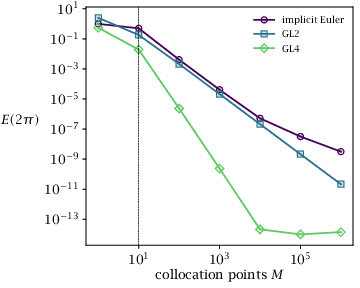## Figure 8.4(a):

### Performance of implicit integration methods on a stiff ODE. Accuracy vs. collocation points.## Code for Figure 8.4(a)

### main.m

```
1
2
3
4
5
6
7
8
9
10
11
12
13
14
15
16
17
18
19
20
21
22
23
24
25
26
27
28
29
30
31
32
33
34
35
36
37
38
39
40
41
42
43
44
45
46
47
48
49
50
51
52
53% Accuracy of numerical integration of a stiff ODE.

% Butcher tableaus (only a and b) for the three methods.
tabs = struct();
tabs.ieu = struct('a', 1, 'b', 1);
tabs.mid = struct('a', 0.5, 'b', 1);
tabs.gl4 = struct('a', [1/4, 1/4 - sqrt(3)/6; 1/4 + sqrt(3)/6, 1/4], ...
'b', [1/2, 1/2]);
tabnames = fieldnames(tabs);

% Run each method for varying number of iterations.
x0 = [1; 0];
f = @stiffode;

% Choose number of function evaluations. Note that the figure in the text goes
% up to M = 10^7, which takes about an hour to run.
Ms = [1, 10, 100, 1000, 10000];

err = struct();
converged = struct();
for n = 1:length(tabnames)
n = tabnames{n};
err.(n) = NaN(size(Ms));
converged.(n) = true(size(Ms));
fprintf('Running %s.\n', n);
for i = 1:length(Ms)
M = Ms(i);
fprintf('  M = %d\n', M);
a = tabs.(n).a;
b = tabs.(n).b;
N = ceil(M/length(b)); % Number of integration steps.
h = 2*pi()/N;
x = x0;
for t = 1:N
[x, okay] = butcherintegration(x, f, h, a, b, 100);
converged.(n)(i) = converged.(n)(i) && okay;
end
err.(n)(i) = norm(x - x0);
end
end

% Make a plot.
figure();
hold('on');
colors = {'r', 'g', 'b'};
for n = 1:length(tabnames)
c = colors{n};
n = tabnames{n};
loglog(Ms, err.(n), ['-o', c], 'displayname', n);
end
xlabel('Collocation points M');
ylabel('Error');
legend('location', 'northwest');

```

### stiffode.m

```
1
2
3
4
5
6
7
8
9
10function [xdot, jac] = stiffode(x)
% [xdot, jac] = stiffode(x)
%
% Example stiff ODE. First output is dx/dt; second is Jacobian of dx/dt.
A = [0 1; -1 0];
lambda = 500;
rho = (x'*x - 1);
xdot = A*x - lambda*rho*x;
jac = A - eye(2)*lambda*rho - 2*lambda*(x*x');
end%function

```

### butcherintegration.m

```
1
2
3
4
5
6
7
8
9
10
11
12
13
14
15
16
17
18
19
20
21
22
23
24
25
26
27
28
29
30
31
32
33
34
35
36
37
38
39
40
41
42
43
44
45
46
47
48
49
50
51
52
53
54
55
56
57
58
59
60
61
62
63
64
65
66
67
68
69
70
71
72
73
74
75
76
77
78
79
80
81
82
83
84
85
86
87
88
89
90
91
92
93function [xplus, converged, iter, k] = butcherintegration(x, func, h, a, b, Niter)
% [xplus, converged, iter, k] = butcherintegration(x, f, h, a, b, [Niter=100])
%
% Performs numerical integration of f starting from x for h time units.
%
% f must be a function handle that returns dx/dt. If a defines an implicit
% method, then f must also return the Jacobian of dx/dt. In either case, f must
% be time-invariant.
%
% a and b should be the coefficients from the Butcher tableau for the
% integration scheme. If the a matrix defines an explicit scheme (i.e., zero on
% and above the diagonal, then the explicit formulas will be used. Otherwise,
% the implicit equations are solved using Newton's method.
%
% Niter is the maximum number of iterations to perform. xguess is a guess for
% x at the next timestep.
narginchk(5, 6);
if nargin() < 6
Niter = 100;
end

% Check arguments.
x = x(:);
Nx = length(x);
b = h*b(:);
Ns = length(b);
if ~isequal(size(a), [Ns, Ns])
error('a must be a square matrix with a column for each element of b!');
end
a = h*a;

% Decide if tableau is explicit or not.
if nnz(triu(a)) == 0
k = butcher_explicit(x, func, a);
converged = true();
iter = 0;
else
[k, converged, iter] = butcher_implicit(x, func, a, Niter);
end

xplus = x + k*b;

end%function

% *****************************************************************************

function k = butcher_explicit(x, func, a)
% Performs an explicit integration step.
Nx = length(x);
Ns = size(a, 1);
k = zeros(Nx, Ns);
for i = 1:Ns
k(:,i) = func(x + k*a(i,:)');
end
end%function

% *****************************************************************************

function [k, converged, iter] = butcher_implicit(x, func, a, Niter)
% Solves implicit integration using Newton's Method. Timestep is assumed 1.
narginchk(4, 4);
Nx = length(x);
Ns = size(a, 1);;
k = zeros(Nx, Ns);
converged = false();
iter = 0;
while ~converged && iter < Niter
iter = iter + 1;

dX = k*a';
if Ns == 1
[f, J] = func(x + dX);
J = a*J;
else
f = zeros(Nx*Ns, 1);
J = zeros(Nx*Ns, Nx*Ns);
for i = 1:Ns
I = ((i - 1)*Nx + 1):(i*Nx);
[f(I), Ji] = func(x + dX(:,i));
J(I,:) = kron(a(i,:), Ji);
end
end

f = f - k(:);
J = J - eye(Nx*Ns);
dk = -J\f;
k = k + reshape(dk, [Nx, Ns]);

converged = norm(dk) < 1e-8;
end

end%function

```